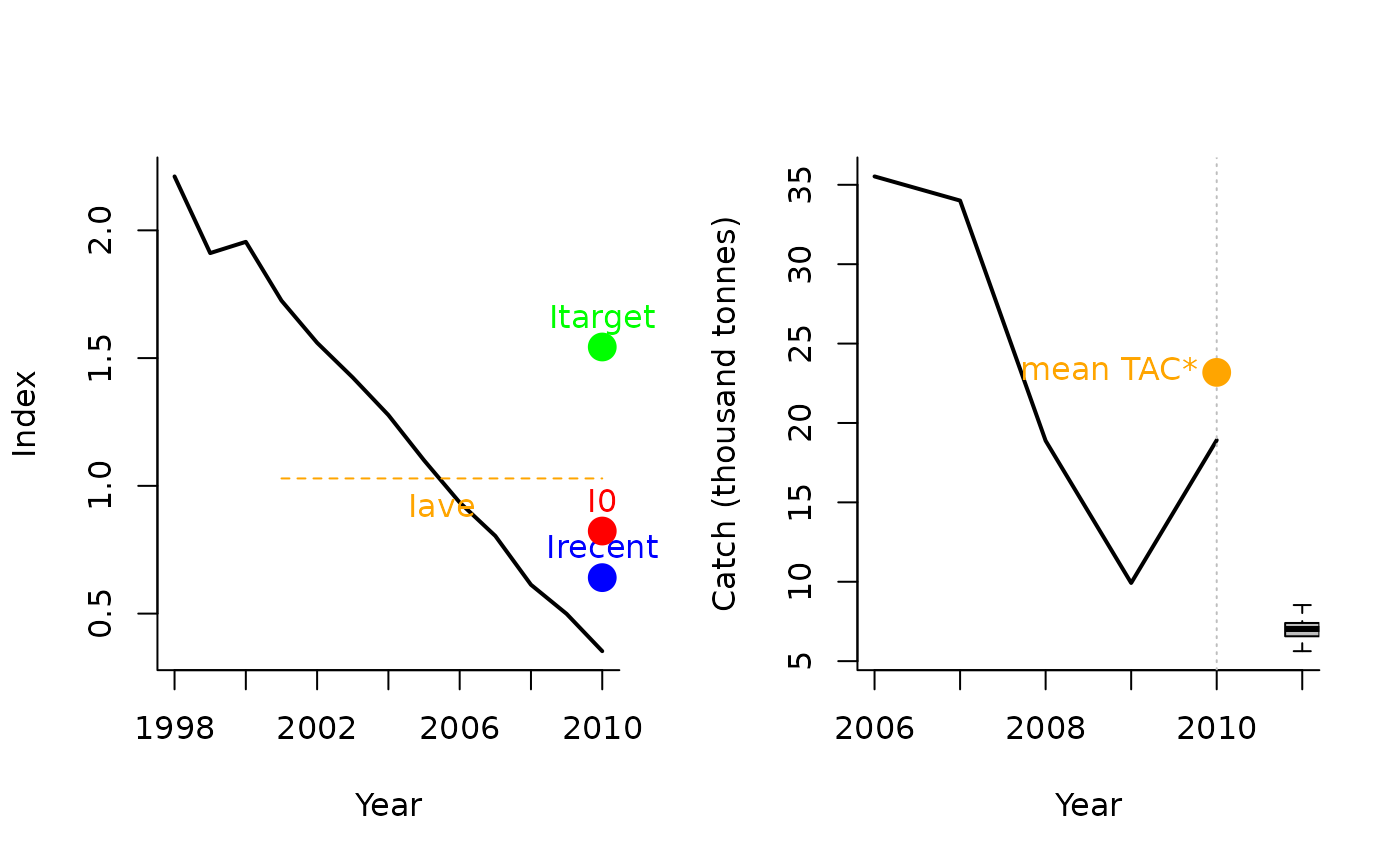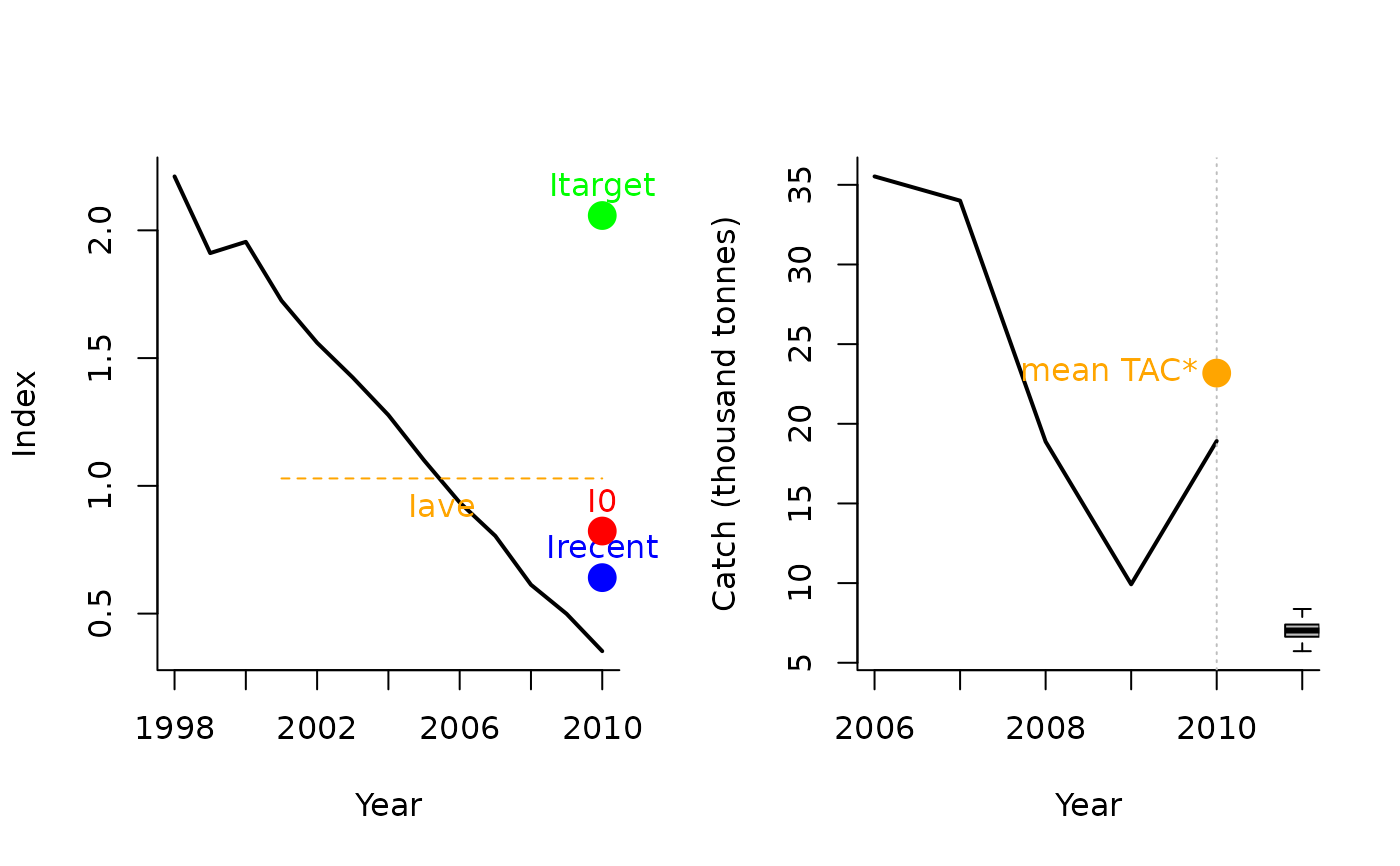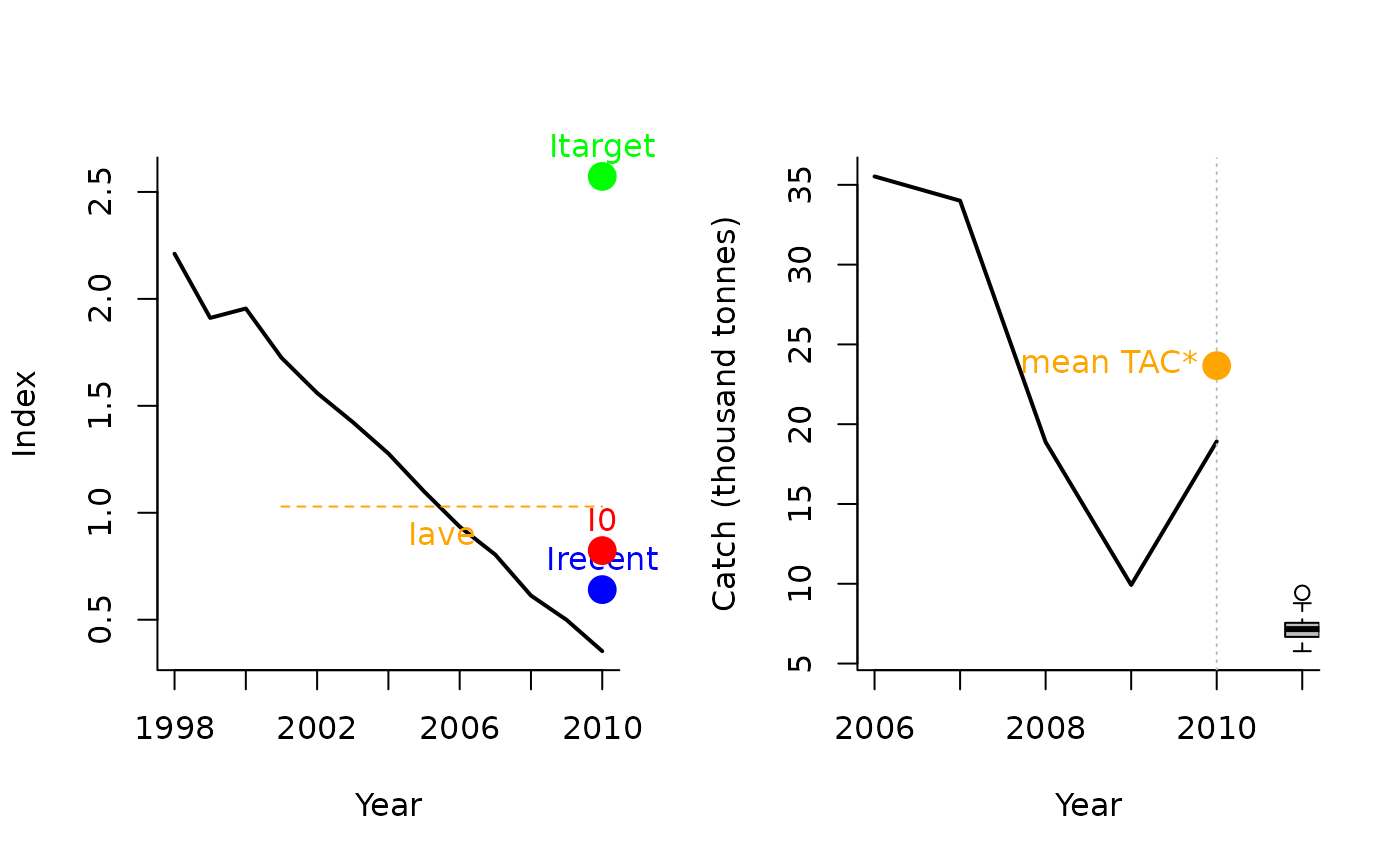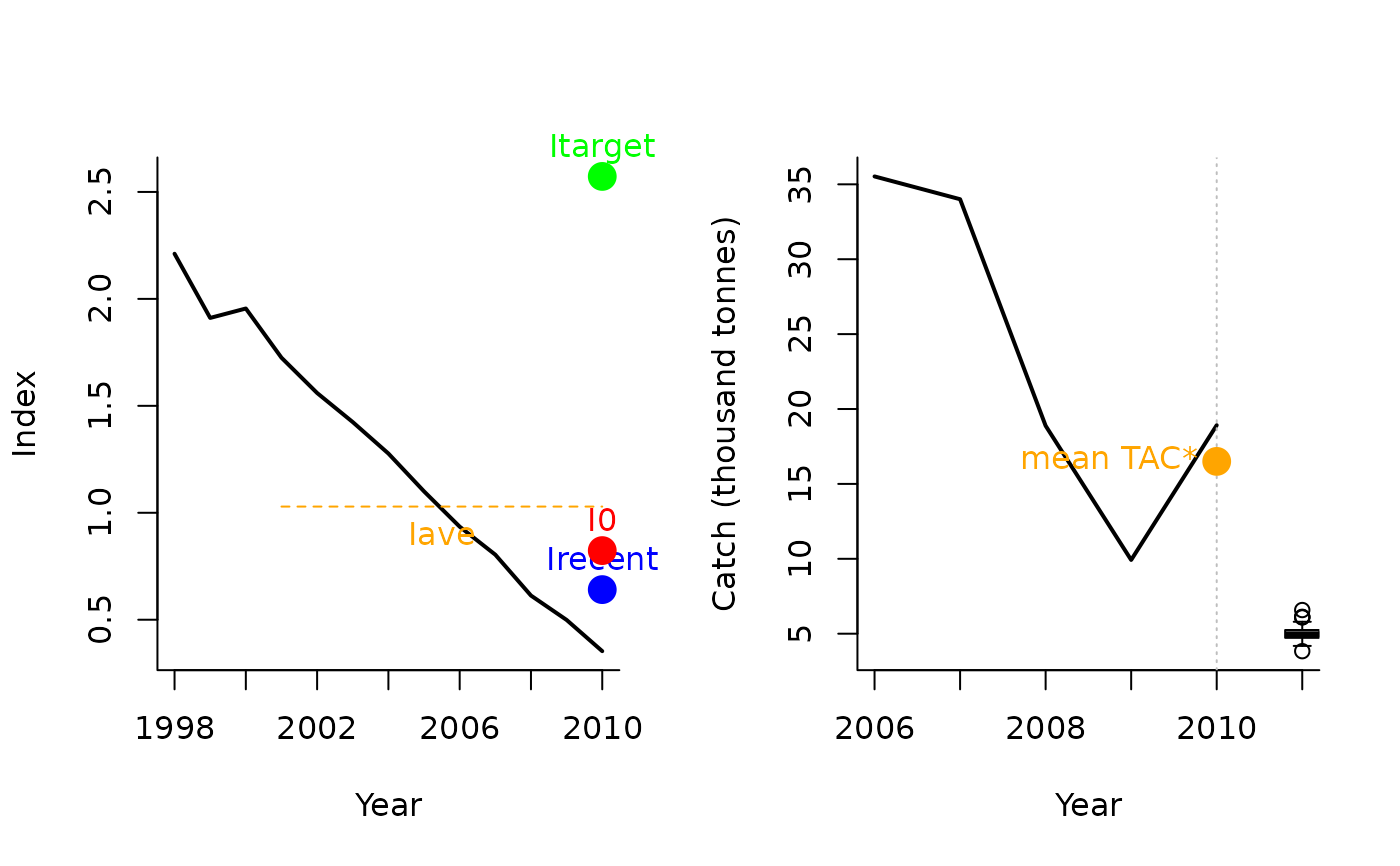A management procedure that incrementally adjusts the TAC (starting from reference level that is a fraction of mean recent catches) to reach a target CPUE / relative abundance index

Itarget1(x, Data, reps = 100, plot = FALSE, yrsmth = 5, xx = 0, Imulti = 1.5)

Itarget2(x, Data, reps = 100, plot = FALSE, yrsmth = 5, xx = 0, Imulti = 2)

Itarget3(x, Data, reps = 100, plot = FALSE, yrsmth = 5, xx = 0, Imulti = 2.5)

Itarget4(x, Data, reps = 100, plot = FALSE, yrsmth = 5, xx = 0.3, Imulti = 2.5)

## Arguments

x

A position in the data object

Data

A data object

reps

The number of stochastic samples of the MP recommendation(s)

plot

Logical. Show the plot?

yrsmth

Years over which the average index is calculated.

xx

Parameter controlling the fraction of mean catch to start using in first year

Imulti

Parameter controlling how much larger target CPUE / index is compared with recent levels.

## Value

An object of class Rec-class with the TAC slot populated with a numeric vector of length reps

## Details

Four index/CPUE target MPs proposed by Geromont and Butterworth 2014. Tested by Carruthers et al. 2015.

The TAC is calculated as: If $$I_\textrm{recent} \geq I_0$$: $$\textrm{TAC}= 0.5 \textrm{TAC}^* \left[1+\left(\frac{I_\textrm{recent} - I_0}{I_\textrm{target} - I_0}\right)\right]$$

else: $$\textrm{TAC}= 0.5 \textrm{TAC}^* \left[\frac{I_\textrm{recent}}{I_0}^2\right]$$

where $$I_0$$ is $$0.8 I_{\textrm{ave}}$$ (the average index over the 2 x yrsmth years prior to the projection period), $$I_\textrm{recent}$$ is the average index over the past yrsmth years, and $$I_\textrm{target}$$ is Imulti times $$I_{\textrm{ave}}$$, and $$\textrm{TAC}^*$$ is: $$(1-x)C$$ where $$x$$ is argument xx and C is the average catch over the last 5 years of the historical period.

## Functions

• Itarget1: The less precautionary TAC-based MP

• Itarget2: Increasing biologically precautionary TAC-based MP

• Itarget3: Increasing biologically precautionary TAC-based MP

• Itarget4: The most biologically precautionary TAC-based MP

## Required Data

See Data-class for information on the Data object

Itarget1: Cat, Ind, LHYear, Year

## Rendered Equations

See Online Documentation for correctly rendered equations

Other Index methods: GB_slope(), GB_target(), Gcontrol(), ICI(), Iratio(), Islope1(), Itarget1_MPA(), ItargetE1()

T. Carruthers

## Examples

Itarget1(1, MSEtool::Atlantic_mackerel, plot=TRUE)#> TAC (median)
#>     6.992851
Itarget2(1, MSEtool::Atlantic_mackerel, plot=TRUE)#> TAC (median)
#>     7.011153
Itarget3(1, MSEtool::Atlantic_mackerel, plot=TRUE)#> TAC (median)
#>     7.082726
Itarget4(1, MSEtool::Atlantic_mackerel, plot=TRUE)#> TAC (median)
#>     4.943079